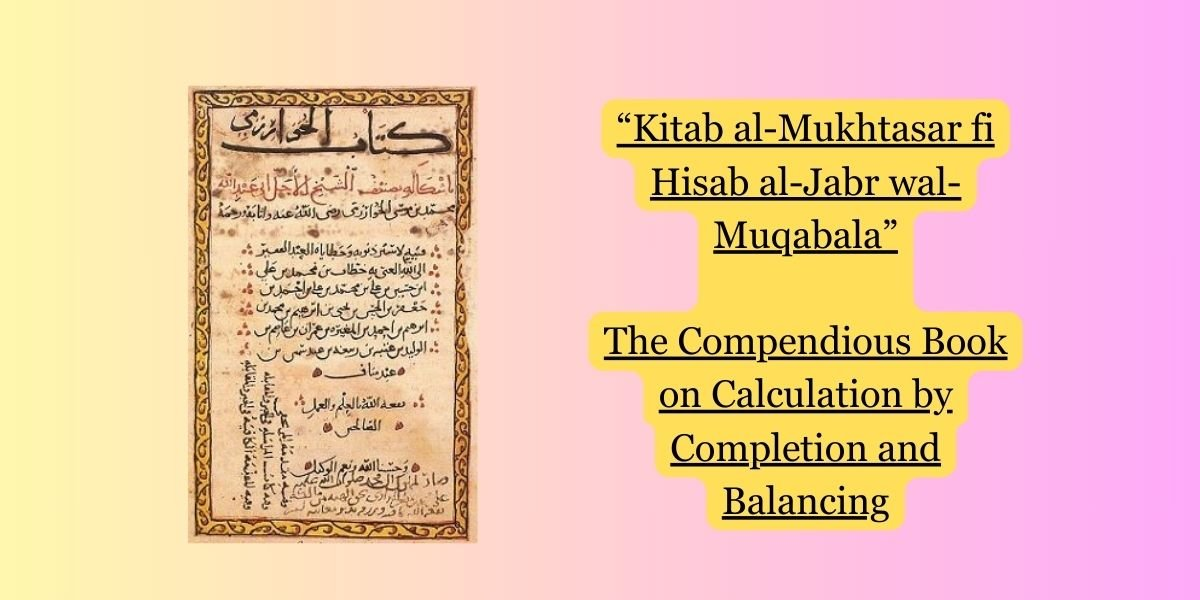“Kitab al-Mukhtasar fi Hisab al-Jabr wal-Muqabala,” translated as “The Compendious Book on Calculation by Completion and Balancing,” is a foundational work by the Persian mathematician and scholar Muhammad ibn Musa al-Khwarizmi. This book, written in the 9th century, is considered one of the most influential mathematical texts in history and is often credited with laying the groundwork for the development of algebra.

### Key Aspects of the Book:

1. Introduction of Algebraic Methods:
• Al-Khwarizmi’s book is primarily concerned with solving quadratic equations and linear equations. It presents systematic methods for solving these equations, marking the beginning of algebra as a distinct branch of mathematics.
2. Al-Jabr and Al-Muqabala:
• The title of the book reflects its content. “Al-Jabr” refers to the process of completing the square, which is a method used to solve quadratic equations. “Al-Muqabala” refers to the process of balancing equations, a key aspect of solving linear equations.
3. Solution Techniques:
• Al-Khwarizmi’s work presents step-by-step methods for solving different types of equations, including both positive and negative roots.
4. Use of Geometric Shapes:
• The book employs geometric shapes to illustrate solutions, making the mathematical concepts more accessible.
5. Decimal System and Numerals:
• Al-Khwarizmi’s book also introduces and popularizes the decimal system and Hindu-Arabic numerals in the Islamic world. This system, which includes the concept of zero, revolutionized mathematical notation and calculation methods.

### Influence and Legacy:

1. Foundation of Algebra:
• “Kitab al-Mukhtasar fi Hisab al-Jabr wal-Muqabala” laid the foundation for the development of algebra as an independent field of study. The word “algebra” itself is derived from the Arabic term “al-jabr” used in the title.
2. Transmission to the West:
• The book was later translated into Latin during the Middle Ages, and its teachings influenced European mathematicians. Al-Khwarizmi’s work played a crucial role in transmitting mathematical knowledge from the Islamic world to medieval Europe.
3. Algorithm and Algorism:
• The term “algorithm” is derived from the Latinization of Al-Khwarizmi’s name. The process described in the book for solving equations using systematic methods contributed to the development of algorithms.